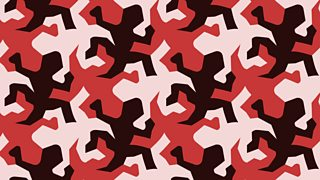# Minimising waste

Using energy and materials responsibly is an important aspect of designing

will lose money if too much scrap is produced during cutting. Profits could be increased if they are able to find ways to lower the cost per product by saving material. Some manufacturers will pass on the savings that they make to the and lower the cost of the product.

shapes can help arrange items to be cut so that, when fitted together, as many as possible can be cut out of a sheet of material.Tessellated shapes

software will automatically fit irregular shapes as close together as possible. A designer could use a package with built-in nesting tools to try different nested shapes and work out what outputs use the least surface area of material.

Example of nesting

are used when cutting fabrics, as fabric has a . This means that it has a different appearance when viewed in another direction, and that pattern pieces cannot be tessellated or nested as effectively as wood, metal or plastic.

## Calculating surface area and volume

Calculating the amount of material needed can be done by using a or by measuring a . Simple measurements can be used to work out the area and volume of shapes. This will help to calculate how much raw material is needed to make a product, as well as how much space would be available inside the product.

### Example

Calculate the surface area of all the sides below, to determine how much timber would be needed to manufacture the jewellery box, and the volume of the finished product. In this example, include the lid in the calculation of each face.

Surface area can be calculated by adding together the areas of the six faces.

First, find the total area of the two different-sized faces:

End panels:

Area = 30 cm × 30 cm = 900 cm2

Total area = 2 × 900 cm2

= 1,800 cm2

Side panels:

Area = 60 cm × 30 cm = 1,800 cm2

Total area = 4 × 1,800 cm2

= 7,200 cm2

Surface area = 1,800 cm2 + 7,200 cm2

= 9,000 cm2

Therefore, 9,000 cm2 of timber is required to construct the jewellery box.

Volume of a prism = area of the × length.

= 900 cm2 × 60 cm

= 54,000 cm3

Question

Calculate how much timber would be needed to manufacture the toy box below, and the volume of the finished product. Again, include the lid in your surface area calculations.

100 cm = 1m

Area of end panel = 1 m × 1 m = 1 m2

Total area of two end panels = 2 × 1 m2 = 2 m2

Area of side panel = 1 m × 4 m = 4 m2

Total area of four side panels = 4 × 4 m2 = 16 m2

Surface area = 2 m2 + 16 m2 = 18 m2 of timber

Volume = 2 m2 × 4 m = 8 m3